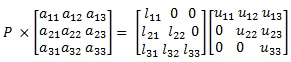# SQL Server LU decomposition

LUdecompN

Updated: 19 January 2015

Use the table-value function LUdecompN to calculate the LU factorization of an N x N matrix A in 3rd normal form using partial pivoting. LUdecompN returns a lower triangular matrix L, an upper triangular matrix U, and a permutation matrix P such that,

LU = PA

This means that L has only zeroes above the diagonal and U has only zeroes below the diagonal.

For a 3 x 3 matrix this becomes:Syntax
SELECT * FROM [wct].[LUdecompN](
<@Matrix_TableName, nvarchar(max),>
,<@Matrix_Key1ColumnName, nvarchar(4000),>
,<@Matrix_Key2ColumnName, nvarchar(4000),>
,<@Matrix_DataColumnName, nvarchar(4000),>
,<@Matrix_GroupedColumnName, nvarchar(4000),>
,<@Matrix_GroupedColumnValue, sql_variant,>)
Arguments
@Matrix_TableName
the name, as text, of the table or view that contains the values in the square (N x N) array to be used in the LUdecompN calculation.
@Matrix_Key1ColumnName
the name, as text, of the column in the table or view specified by @Matrix_TableName that contains the ‘row number’ value used in the array.
@Matrix _Key2ColumnName
the name, as text, of the column in the table or view specified by @Matrix _TableName that contains the ‘column number’ value used in the array.
@Matrix _DataColumnName
the name, as text, of the column in the table or view specified by @Matrix _TableName that contains the matrix values to be used in the product. Data returned from the @Matrix_DataColumnName must be of the type float or of a type that implicitly converts to float.
@Matrix_GroupedColumnName
the name, as text, of the column in the table or view specified by @Matrix_TableName which will be used for grouping the results.
@Matrix_GroupedColumnValue
the column value to do the grouping on.
Return Types
TABLE (
[RowNum] [int] NULL,
[ColNum] [int] NULL,
[Value] [float] NULL,
[Type] [nvarchar](2) NULL
)
Remarks
·         The number of columns in the matrix must be equal to the number of rows or an error will be returned.
·         Use the LUdecompN_q function for more complicated queries.
·         Use LUdecomp for a table not in third-normal form.
·         The function returns an error if the matrix contains a non-numeric value.
·         The returned Type column contains 'L', 'U', or 'P'
Examples
In this example, we will populate a temporary table #m and calculate its LU factorization.
SELECT
*
INTO
#m
FROM (VALUES
(0,0,0.002),
(0,1,1.231),
(0,2,2.471),
(1,0,1.196),
(1,1,3.165),
(1,2,2.54),
(2,0,1.475),
(2,1,4.271),
(2,2,2.142)
) m(r,c,x)

SELECT
*
FROM
wct.LUdecompN('#m','r','c','x','',NULL)

This produces the following result.
RowNum      ColNum      Value                  Type
----------- ----------- ---------------------- ----
0           0           1                      L
0           1           0                      L
0           2           0                      L
1           0           0.00167224080267559    L
1           1           1                      L
1           2           0                      L
2           0           1.23327759197324       L
2           1           0.299970803835884      L
2           2           1                      L
0           0           1.196                  U
0           1           3.165                  U
0           2           2.54                   U
1           0           0                      U
1           1           1.22570735785953       U
1           2           2.4667525083612        U
2           0           0                      U
2           1           0                      U
2           2           -1.73047881640933      U
0           0           0                      P
0           1           1                      P
0           2           0                      P
1           0           1                      P
1           1           0                      P
1           2           0                      P
2           0           0                      P
2           1           0                      P
2           2           1                      P

Note that the results are returned in third-normal form. If we wanted to a more traditional (de-normalized) presentation of the results, we can us the PIVOT function.
SELECT
Type,,,
FROM (
SELECT
*
FROM
wct.LUdecompN('#m','r','c','x','',NULL)
)d
PIVOT(SUM(Value) for ColNum in(,,)) as P

This produces the following result.
Type 0                      1                      2
---- ---------------------- ---------------------- ----------------------
L    1                      0                      0
L    0.00167224080267559    1                      0
L    1.23327759197324       0.299970803835884      1
P    0                      1                      0
P    1                      0                      0
P    0                      0                      1
U    1.196                  3.165                  2.54
U    0                      1.22570735785953       2.4667525083612
U    0                      0                      -1.73047881640933

In this example, we demonstrate how to reconstruct the input matrix using the calculation P'LU.
SELECT
k.*
FROM (
SELECT
Type as MatrixType,
wct.NMATRIX2STRING(RowNum, ColNum, Value) as Matrix
FROM
wct.LUdecompN('#m','r','c','x','',NULL)
GROUP BY
Type
) p PIVOT(MAX(Matrix) FOR MatrixType IN(L,P,U))d
CROSS APPLY
wct.MATRIX(wct.MATMULT(wct.TRANSPOSE(P),wct.MATMULT(L,U)))K

This produces the following result.
RowNum      ColNum      ItemValue
----------- ----------- ----------------------
0           0           0.00200000000000001
0           1           1.231
0           2           2.471
1           0           1.196
1           1           3.165
1           2           2.54
2           0           1.475
2           1           4.27099999999998
2           2           2.14199999999999

### SupportCopyright 2008-2023 Westclintech LLC         Privacy Policy        Terms of Service